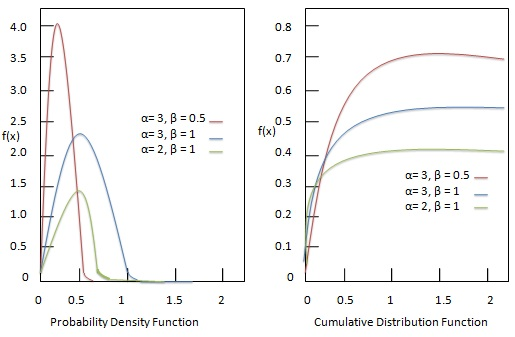# Statistics - Inverse Gamma Distribution

Inverse Gamma Distribution is a reciprocal of gamma probability density function with positive shape parameters ${\alpha, \beta }$ and location parameter ${ \mu }$. ${\alpha }$ controls the height. Higher the ${\alpha }$, taller is the probability density function (PDF). ${\beta }$ controls the speed. It is defined by following formula.

## Formula

${ f(x) = \frac{x^{-(\alpha+1)}e^{\frac{-1}{\beta x}}}{ \Gamma(\alpha) \beta^\alpha} \\[7pt] \, where x \gt 0 }$

Where −

• ${\alpha}$ = positive shape parameter.

• ${\beta}$ = positive shape parameter.

• ${x}$ = random variable.

Following diagram shows the probability density function with different parameter combinations.## Useful Video Courses

Video

#### Class 11th Statistics for Economics

40 Lectures 3.5 hours

Video

#### Statistics

40 Lectures 2 hours

Video

#### Applied Statistics in Python for Machine Learning Engineers

66 Lectures 1.5 hours

Video

#### An Introduction to Wait Statistics in SQL Server

22 Lectures 1 hours

Video

#### Geospatial Data Science: Statistics and Machine Learning

60 Lectures 12 hours

Video

#### Basic Statistics & Regression for Machine Learning in Python

65 Lectures 5 hours